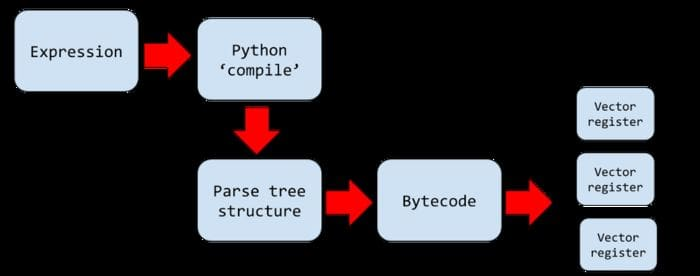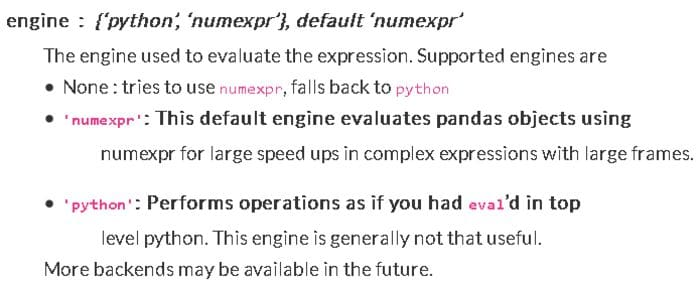# Speed up your Numpy and Pandas with NumExpr PackageImage source: Pixabay and author made collage

### Introduction

Numpy and Pandas are probably the two most widely used core Python libraries for data science (DS) and machine learning (ML)tasks. Needless to say, the speed of evaluating numerical expressions is critically important for these DS/ML tasks and these two libraries do not disappoint in that regard.

Under the hood, they use fast and optimized vectorized operations (as much as possible) to speed up the mathematical operations. Plenty of articles have been written about how Numpy is much superior (especially when you can vectorize your calculations) over plain-vanilla Python loops or list-based operations.

How Fast Numpy Really is and Why?
A comparison with standard Python Lists

Data science with Python: Turn your conditional loops to Numpy vectors
It pays to even vectorize conditional loops for speeding up the overall data transformation.

In this article, we show, how using a simple extension library, called ‘NumExpr’, one can improve the speed of the mathematical operations, which the core Numpy and Pandas yield.

### Let’s see it in the action

The example Jupyter notebook can be found here in my Github repo.

### Install the NumExpr library

First, we need to make sure we have the library `numexpr`. So, as expected,

The project is hosted here on Github. It is from the PyData stable, the organization under NumFocus, which also gave rise to Numpy and Pandas.

As per the source, “NumExpr is a fast numerical expression evaluator for NumPy. With it, expressions that operate on arrays, are accelerated and use less memory than doing the same calculation in Python. In addition, its multi-threaded capabilities can make use of all your cores — which generally results in substantial performance scaling compared to NumPy.” (source)

Here is the detailed documentation for the library and examples of various use cases.

### A simple vector-scalar operation

We start with the simple mathematical operation — adding a scalar number, say 1, to a Numpy array. For using the NumExpr package, all we have to do is to wrap the same calculation under a special method `evaluate` in a symbolic expression. The following code will illustrate the usage clearly,Wow! That was magical! All we had to do was to write the familiar `a+1` Numpy code in the form of a symbolic expression `"a+1"` and pass it on to the `ne.evaluate()` function. And we got a significant speed boost — from 3.55 ms to 1.94 ms on average.

Note that we ran the same computation 200 times in a 10-loop test to calculate the execution time. Now, of course, the exact results are somewhat dependent on the underlying hardware. You are welcome to evaluate this on your machine and see what improvement you got.

### Arithmetic involving two arrays

Let’s dial it up a little and involve two arrays, shall we? Here is the code to evaluate a simple linear expression using two arrays,That is a big improvement in the compute time from 11.7 ms to 2.14 ms, on the average.

### A somewhat complex operation involving more arrays

Now, let’s notch it up further involving more arrays in a somewhat complicated rational function expression. Suppose, we want to evaluate the following involving five Numpy arrays, each with a million random numbers (drawn from a Normal distribution),Here is the code. We create a Numpy array of the shape (1000000, 5) and extract five (1000000,1) vectors from it to use in the rational function. Also note, how the symbolic expression in the NumExpr method understands ‘sqrt’ natively (we just write `sqrt`).Whoa! That shows a huge speed boost from 47 ms to ~ 4 ms, on average. In fact, this is a trend that you will notice that the more complicated the expression becomes and the more number of arrays it involves, the higher the speed boost becomes with Numexpr!

### Logical expressions/ Boolean filtering

As it turns out, we are not limited to the simple arithmetic expression, as shown above. One of the most useful features of Numpy arrays is to use them directly in an expression involving logical operators such as `>` or `<` to create Boolean filters or masks.

We can do the same with NumExpr and speed up the filtering process. Here is an example where we check whether the Euclidean distance measure involving 4 vectors is greater than a certain threshold.This kind of filtering operation appears all the time in a data science/machine learning pipeline, and you can imagine how much compute time can be saved by strategically replacing Numpy evaluations by NumExpr expressions.

### Complex numbers!

We can make the jump from the real to the imaginary domain pretty easily. NumExpor works equally well with the complex numbers, which is natively supported by Python and Numpy. Here is an example, which also illustrates the use of a transcendental operation like a logarithm.### Impact of the array size

Next, we examine the impact of the size of the Numpy array over the speed improvement. For this, we choose a simple conditional expression with two arrays like `2*a+3*b < 3.5` and plot the relative execution times (after averaging over 10 runs) for a wide range of sizes. The code is in the Notebook and the final result is shown below,Image source: Author code generated

### Pandas “eval” method

This is a Pandas method that evaluates a Python symbolic expression (as a string). By default, it uses the NumExpr engine for achieving significant speed-up. Here is an excerpt of from the official doc,We show a simple example with the following code, where we construct four DataFrames with 50000 rows and 100 columns each (filled with uniform random numbers) and evaluate a nonlinear transformation involving those DataFrames — in one case with native Pandas expression, and in other case using the `pd.eval()` method.### Impact of the DataFrame size

We do a similar analysis of the impact of the size (number of rows, while keeping the number of columns fixed at 100) of the DataFrame on the speed improvement. The result is shown below,Image source: Author code generated

### How it works and supported operators

The details of the manner in which Numexpor works are somewhat complex and involve optimal use of the underlying compute architecture. You can read about it here.

### A short explanation

Basically, the expression is compiled using Python `compile` function, variables are extracted and a parse tree structure is built. This tree is then compiled into a Bytecode program, which describes the element-wise operation flow using something called ‘vector registers’ (each 4096 elements wide). The key to speed enhancement is Numexpr’s ability to handle chunks of elements at a time.It skips the Numpy’s practice of using temporary arrays, which waste memory and cannot be even fitted into cache memory for large arrays.

Also, the virtual machine is written entirely in C which makes it faster than native Python. It is also multi-threaded allowing faster parallelization of the operations on suitable hardware.

### Supported operators

NumExpr supports a wide array of mathematical operators to be used in the expression but not conditional operators like `if` or `else`. The full list of operators can be found here.

You can also control the number of threads that you want to spawn for parallel operations with large arrays by setting the environment variable `NUMEXPR_MAX_THREAD`. Currently, the maximum possible number of threads is 64 but there is no real benefit of going higher than the number of virtual cores available on the underlying CPU node.

### Summary

In this article, we show how to take advantage of the special virtual machine-based expression evaluation paradigm for speeding up mathematical calculations in Numpy and Pandas. Although this method may not be applicable for all possible tasks, a large fraction of data science, data wrangling, and statistical modeling pipeline can take advantage of this with minimal change in the code.

Also, you can check the author’s GitHub repositories for code, ideas, and resources in machine learning and data science. If you are, like me, passionate about AI/machine learning/data science, please feel free to add me on LinkedIn or follow me on Twitter.

Bio: Tirthajyoti Sarkar is Sr Principal Engineer at ON Semiconductor.

Original. Reposted with permission.

Related: